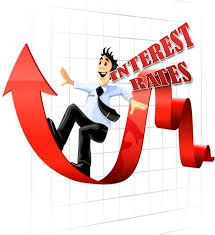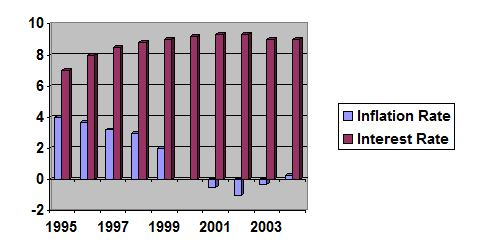Economics

# Relation between Inflation and Interest RateThe relation of inflation and interest rate can be described the term controller and controlled, where interest rate is controller and inflation is controlled. That means inflation is controlled by the interest rate. To cheek the inflation by the control of credit flow in the private sector of the country Bangladesh Bank follows various type of method like: –

•  Bank rate policy
•  Open market operation
•  Variable reserve ratio
•  Selective credit control
•  Direct action
•  Moral persuasion
•  Publicity
•  Fixation of margin requirements

Among those methods, the rate of interest rate that means Bank rate policy is one of the popular methods. Under these method all kind of interest rate, that means interest rate on deposit and interest rate on credit also increase. These methods governed that; the rate at which commercial banks were prepared to make advances to customers and the rate they paid on deposits. Thus if the monetary authorities wished to rise or lower interest rates generally this could be achieved by rising of lowering bank. If there was a tendency towards inflation in the economy, bank rate could be raised in order to make borrowing from the banks dearer. This would reduce somewhat the demand of businessman for bank advances. Since some forms of economic activity which previously were profitable would no longer be so. To some extent, therefore, production would be curtailed and since the reduced bank lending would cause a contraction of bank deposits, the total volume of purchasing power would be reduced and inflation would tend to disappear. Here I prepared a assumption based table and show a graph to show the impact of interest rate in inflation of a country: –

 Year Interest rate Inflation rate 1995 7 4 1996 8 3.7 1997 8.5 3.2 1998 8.8 3 1999 9 2 2000 9.2 -.8 2001 9.3 -.5 2002 9.3 -1 2003 9 -.3 2004 9 .3

Table:- Assumption Based inflation rate and interest rate relationFigure: Assumption Based relationship between interest rate and inflation

In above table and graph we show a assumption based relation between the inflation and interest rate. Here we see that if interest rate is increase inflation rate is decrease and if interest rate is decrease then the inflation rate is increase. This is happened because if interest rate is increase the business man don’t want to borrow from the bank as a result the activity of production is decrease and money circulation in the open market also decrease as a result money value is increase that means decrease in inflation rate. And if interest is decrease then the different thing is happen.